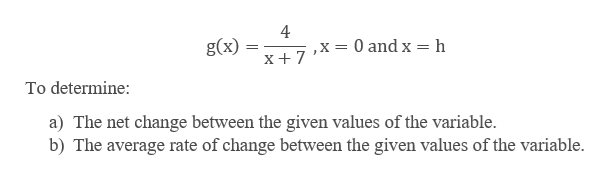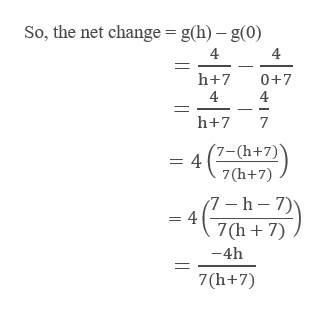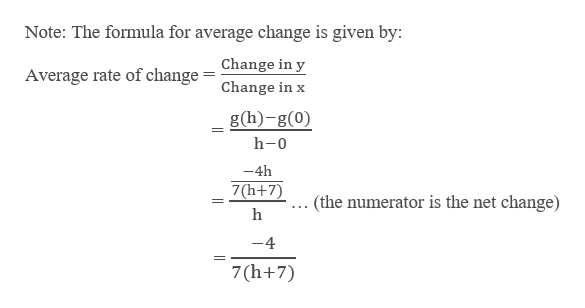# A function is given. g(x) = 4/(x+7); x=0, x=h(a) Determine the net change between the given values of the variable.(b) Determine the average rate of change between the given values of the variable.

Question
63 views

A function is given.

g(x) = 4/(x+7); x=0, x=h

(a) Determine the net change between the given values of the variable.

(b) Determine the average rate of change between the given values of the variable.

check_circle

Step 1

Given a function:help_outlineImage Transcriptionclose4 g(x) x7X= 0 and x = h_ To determine: a) The net change between the given values of the variable b) The average rate of change between the given values of the variable fullscreen
Step 2

We begin with finding the net change of g(x):

Note: Let f (x) be a function continuous on an interval containing a and b with a < b. The net change of f (x) as x
increases from a to b is the difference f (b) – f (a).help_outlineImage TranscriptioncloseSo, the net change = g(h) - g(0) 4 4 h+7 4 0+7 h+7 7 (7-(h+7) 4 7 (h+7) 7 - h 7) = 4 7(h7) -4h 7(h+7) fullscreen
Step 3

Now, we find the Average ra...help_outlineImage TranscriptioncloseNote: The formula for average change is given by: Average rate of change = Change in y Change in x g(h)-g(0) h-0 -4h 7(h+7) . (the numerator is the net change) h -4 7(h+7) fullscreen

### Want to see the full answer?

See Solution

#### Want to see this answer and more?

Solutions are written by subject experts who are available 24/7. Questions are typically answered within 1 hour.*

See Solution
*Response times may vary by subject and question.
Tagged in

### Other